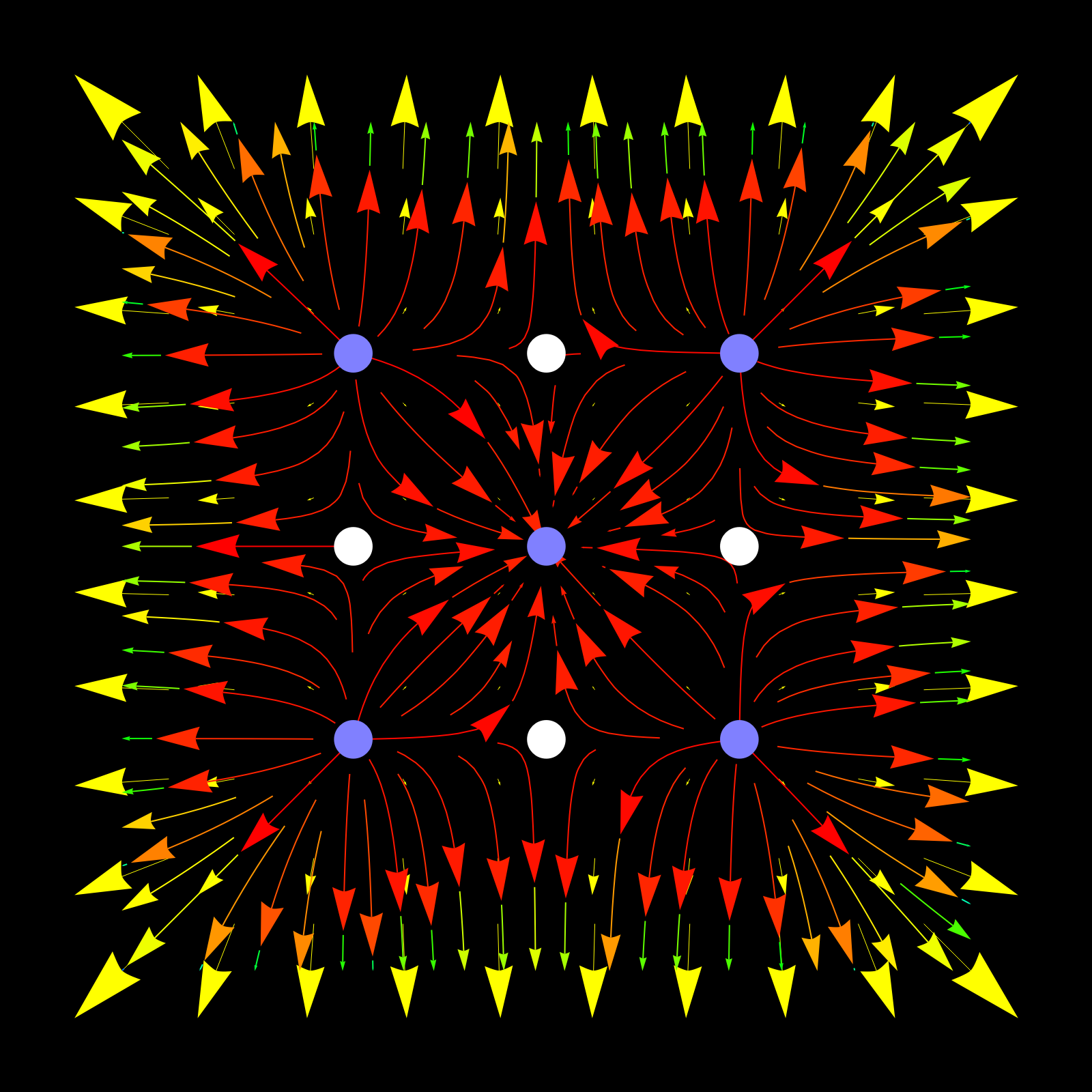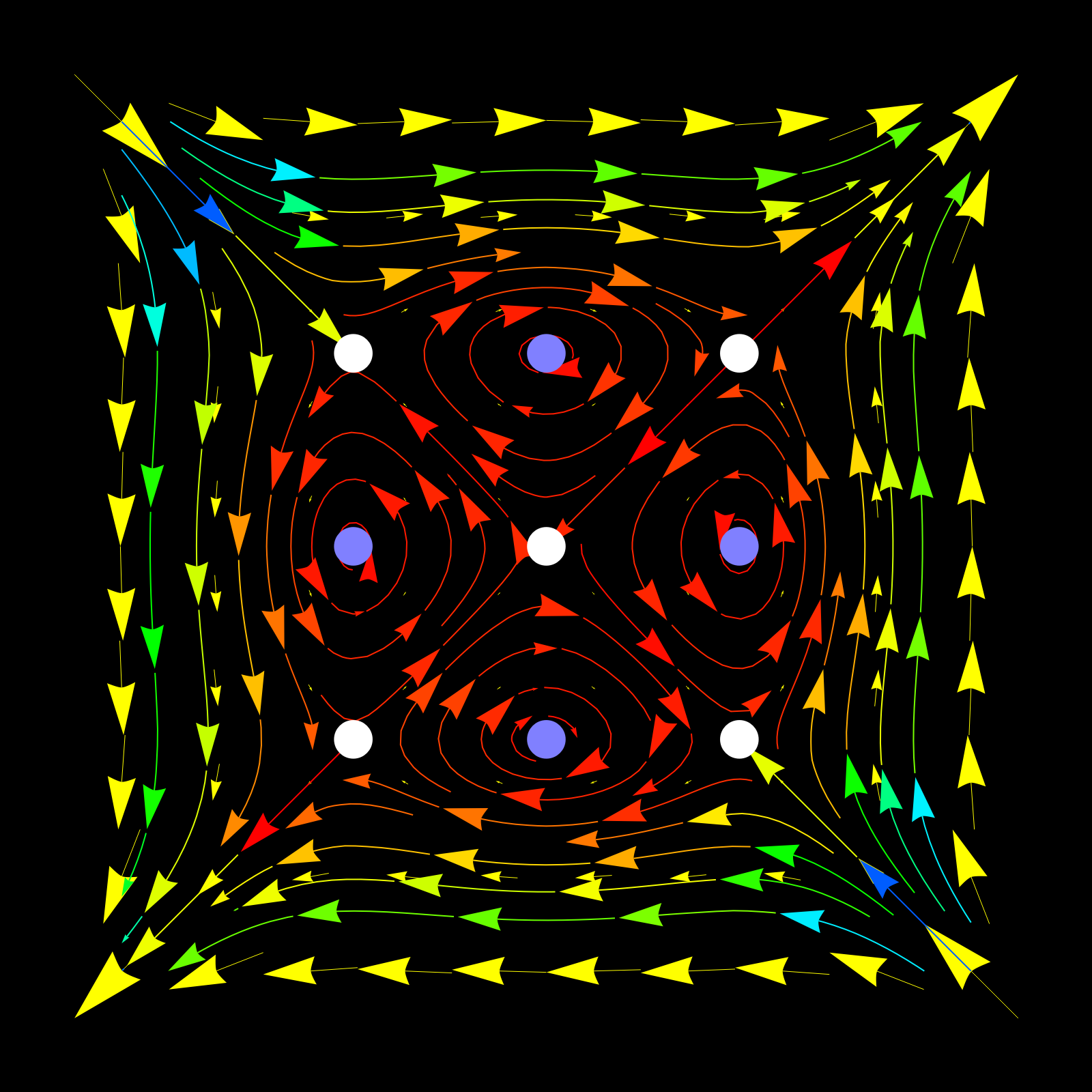# Two vector Fields

## F = [x3 - x, y3 - y ]

This is a gradient field. You can see that from the formula or that Qx - Py = 0. A potential is f(x,y) = x4/4 - x2/2 + y4/4 - xy/2.## F = [y3 - y, x3 - x ]

This is not a gradient field. You see that the curl Qx - Py = 3x^2-3y^2 is nonzero. You see that if x is bigger than y, then the curl is positive and you see the vector field turning counter clockwise there.. If y is bigger than x, you see that the curl is negative. The vector field turns clockwise.